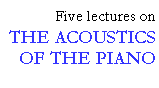## Decay of the piano tone

```
```

As explained by Gabriel Weinreich in the preceding lecture, the decay of a piano tone is complex. The early decay, the so-called prompt sound, is of major importance in judging the tone quality. Therefore it seems reasonable to measure the decay time at the beginning of the note, from the maximum level to -20 dB (T20). This decay should depend mainly on the soundboard, more specifically on the impedance matching between the string and the soundboard motion in the vertical direction. This is so, since the initial blow of the hammer sets the string in vibration mainly in this direction.

To test this hypothesis, a complete assembly with frame, soundboard and plate was fitted with a single string at a few measuring points. The impedance matching was varied by tuning the string to frequencies at and close to a soundboard resonance (see Fig. 10). The change in the impedance matching was registered by observing the change in the decay time of the fundamental when the string was plucked. Starting with a string on the treble bridge, the string was first tuned to D4 = 294 Hz (point 1 in Fig. 10, top) and the string tension was then slowly let down to 210 Hz (point 3). By means of these detunings, we were able to compare the decay times at peaks, valleys and at intermediate points with the same impedance value. Of the two intermediate points, one is located on an upward slope of the curve corresponding to a positive phase angle (point 5), while the other is on a downward slope with a negative phase angle (point 4).

```
```Fig. 10. Changing the impedance matching between string and soundboard by detuning; treble bridge MP 8 (top), and bass bridge MP 12 (bottom). Numbered points indicate the successive frequencies to which a single string was tuned.

```
```

From these experiments, a number of observations were made. As expected, the decay time for the string vibrations is considerably longer at a peak in the impedance curve (off resonance), than in a valley (at resonance). Further, the decay is longer when the phase angle is positive than when the phase angle is negative. Hence, a good match between the string and the soundboard is found when the input impedance is high and the phase angle is positive. The same results were obtained for a string on the bass bridge (see Fig. 10, bottom).

Sharp valleys (resonances) in the impedance curve exert the strongest negative influence on the decay time. Consequently, one characteristic of a high quality soundboard is that the impedance curve exhibit as few dips as possible, avoiding sharp zigzagging. In addition, the overall level of the impedance curve should be high enough to ensure sufficient reflexion of the string energy at the bridge, resulting in a long decay time.

The decay times for the notes on a small upright piano are plotted in Fig. 11. As can be seen, the decay times vary drastically among the notes. In order to judge the perceptual consequences of the irregular curve we may compare two neighbouring notes, F#4 and G4. From the graph, we obtain the corresponding T20-values as 3.5 and 0.7 s, respectively. This large difference in decay time (5:1) suggests an aggravating difference in sustain between these two adjacent notes.

```
```Fig. 11. Decay times (T20) for the notes of a small upright piano, corresponding to a 20 dB drop from maximum sound level

```
```

Another example of the consequences of the irregular decay times of this particular piano is the differences between the two chords C3 - C4 - G4 (3.8, 1.7, 0.7 s) and D3 - D4 - A4 (3.4, 3.3, 2.3 s). These relations between the decay times mean that the first chord will consist mainly of the lowest note (C3) shortly after the attack, since the two higher notes will fade away very quickly. In contrast, the spectrum of the second chord will not change as fast, sounding brighter and fuller compared with the first chord.

This lecture is one of Five lectures on the Acoustics of the piano